### Home > CA > Chapter Ch12 > Lesson 12.1.1 > Problem12-6

12-6.
1. Solve the following equations for x. Homework Help ✎

1. 4x − 6y = 20

2.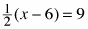3.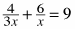4.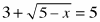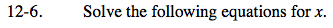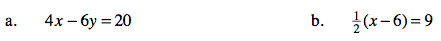Add 6y to both sides and solve for x.

x = 5 + 1.5y

Multiply both sides by 2 first.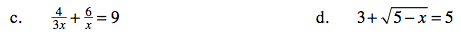Subtract 3 from both sides and thensquare both sides to remove the square root. Solve for x.